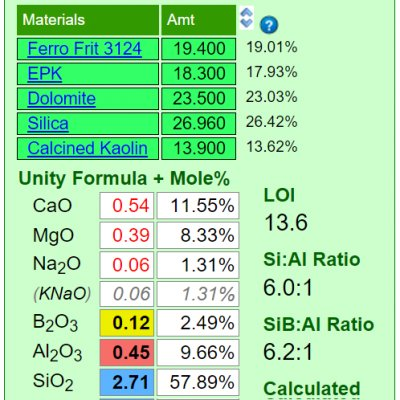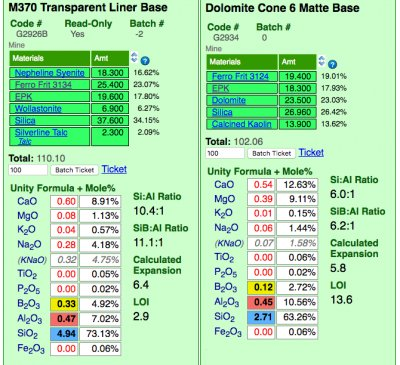Monthly Tech-Tip from Tony Hansen SignUp No tracking! No ads!

# Mole%

Mole% is a way of expressing the oxide formula of a fired glaze or glass. It is a preferred over the formula by many technicians who use glaze or glass chemistry.

## Details

Mole% is a way of expressing the oxide formula of a fired glaze or glass (technicians can extrapolate fired properties like melting temperature, thermal expansion, hardness, resistance to leaching, etc. by examining the chemistry of a glaze). Mole% is preferred over the Seger unity formula by many technicians who use glaze or glass chemistry. Mole% is one of the calculation types in Digitalfire Insight software and Insight-live.com.

Mole% expresses the percentage of oxide molecules by number (as opposed to an analysis which compares their weights). The Seger Unity formula and the Mole% formula are both simply retotals of a raw formula. Consider a raw formula as simply a tabulation of the total number of molecules of each oxide type in a sample. The formulas of batch recipes of different totals therefore also have different totals. Mole% is an expression of the percentage of each oxide type. A Seger Unity formula retotals such that all the fluxes total one. Thus Seger formulas have small numbers, all are less than one except for the SiO2.

When one works with unity formulas in glaze chemistry, changes to a recipe often change only some of the numbers (or only one) in the formula. For example, if the silica weight is adjusted, only the SiO2 amount in the formula will change. However with Mole% all of the numbers change with any change in the recipe. Thus Mole% is more about seeing the concentrations of oxides in the whole than about comparing their concentrations.

Following is an example of how to convert a raw formula to a Mole% formula. The formula is simply totaled and each number then divided by that total times 100.

```           Raw                   Mole
Oxides     Formula               Percent
-----------------------------------------
K2O         0.6  /  12.1 x 100  =  5.0%
CaO         1.3  /  12.1 x 100  = 10.7
MgO         0.2  /  12.1 x 100  =  1.7
ZnO         0.1  /  12.1 x 100  =  0.8
Al2O3       0.9  /  12.1 x 100  =  7.4
SiO2        9.0  /  12.1 x 100  = 74.3
-----                  -----
Total      12.1                  100.0```

## Related Information

### A Limitation of the Seger Unity Formula

Tap picture for full size and resolutionMineral sources of oxides impose their own melting patterns and when one is substituted for another to supply an oxide a different system with its own relative chemistry is entered. An extreme example of this would be to source Al2O3 to a glaze using calcined alumina instead of kaolin. Although the formula may be exactly the same, the fired result would be completely different because very little of the alumina would dissolve into the glaze melt. At the opposite extreme, a different frit could be used to supply a set of oxides (while maintaining the overall chemistry of the glaze) and the fired result would be much more chemically predictable. Why? Because the readily and release their oxides the the melt.

### Insight-Live comparing a glossy and matte cone 6 base glaze recipe

Tap picture for full size and resolutionInsight-live is calculating the unity formula and mole% formula for the two glazes. Notice how different the formula and mole% are for each (the former compares relative numbers of molecules, the latter their weights). The predominant oxides are very different. The calculation is accurate because all materials in the recipe are linked (clickable to view to the right). Notice the Si:Al Ratio: The matte is much lower. Notice the calculated thermal expansion: The matte is much lower because of its high levels of MgO (low expansion) and low levels of KNaO (high expansion). Notice the LOI: The matte is much higher because it contains significant dolomite.

 By Tony Hansen